NEET  >  31 Years NEET Previous Year Questions: Electric Charges & Fields

# 31 Years NEET Previous Year Questions: Electric Charges & Fields

Test Description

## 37 Questions MCQ Test Physics Class 12 | 31 Years NEET Previous Year Questions: Electric Charges & Fields

31 Years NEET Previous Year Questions: Electric Charges & Fields for NEET 2023 is part of Physics Class 12 preparation. The 31 Years NEET Previous Year Questions: Electric Charges & Fields questions and answers have been prepared according to the NEET exam syllabus.The 31 Years NEET Previous Year Questions: Electric Charges & Fields MCQs are made for NEET 2023 Exam. Find important definitions, questions, notes, meanings, examples, exercises, MCQs and online tests for 31 Years NEET Previous Year Questions: Electric Charges & Fields below.
Solutions of 31 Years NEET Previous Year Questions: Electric Charges & Fields questions in English are available as part of our Physics Class 12 for NEET & 31 Years NEET Previous Year Questions: Electric Charges & Fields solutions in Hindi for Physics Class 12 course. Download more important topics, notes, lectures and mock test series for NEET Exam by signing up for free. Attempt 31 Years NEET Previous Year Questions: Electric Charges & Fields | 37 questions in 74 minutes | Mock test for NEET preparation | Free important questions MCQ to study Physics Class 12 for NEET Exam | Download free PDF with solutions
 1 Crore+ students have signed up on EduRev. Have you?
31 Years NEET Previous Year Questions: Electric Charges & Fields - Question 1

### Point charges + 4q, –q and +4q are kept on theX-axis at points x = 0, x =a and x = 2a respectively.

Detailed Solution for 31 Years NEET Previous Year Questions: Electric Charges & Fields - Question 1

Net force on each of the charge due to the other charges is zero. However, disturbance in any direction other than along the line on which the charges lie, will not make the charges return.

31 Years NEET Previous Year Questions: Electric Charges & Fields - Question 2

### A charge q is placed at the centre of the line joining two exactly equal positive charges Q. The system of three charges will be in equilibrium, if q is equal to

Detailed Solution for 31 Years NEET Previous Year Questions: Electric Charges & Fields - Question 2

At equilibrium, net force is zero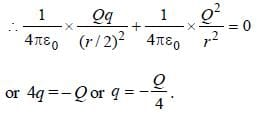31 Years NEET Previous Year Questions: Electric Charges & Fields - Question 3

### An electric dipole, consisting of two opposite charges of 2 × 10-6 C each separated by a distance 3 cm is placed in an electric field of 2 × 105 N/C. Torque acting on the dipole is    

Detailed Solution for 31 Years NEET Previous Year Questions: Electric Charges & Fields - Question 3

Charges (q) = 2 × 10-6 C,

Distance (d) = 3 cm = 3 × 10-2 m

and electric field (E) = 2 × 105 N/C. Torque (t) = q.d.

E = (2 × 10-6) × (3 × 10-2) × (2 × 105)

= 12 × 10-3N-m.

31 Years NEET Previous Year Questions: Electric Charges & Fields - Question 4

There is an electric field E in x-direction. If the work done on moving a charge of 0.2 C through a distance of 2 m along a line making an angle 60° with x-axis is 4J, then what is the value of E?       

Detailed Solution for 31 Years NEET Previous Year Questions: Electric Charges & Fields - Question 4

Charge (q) = 0.2 C; Distance (d) = 2m; Angle
θ = 60º and work done (W) = 4J.
Work done in moving the charge (W)
= F.d cos θ = qEd cos θ

or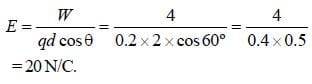31 Years NEET Previous Year Questions: Electric Charges & Fields - Question 5

Intensity of an electric field (E) depends on distance r, due to a dipole, is related as 

Detailed Solution for 31 Years NEET Previous Year Questions: Electric Charges & Fields - Question 5

Intensity of electric field due to a Dipole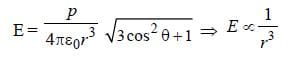31 Years NEET Previous Year Questions: Electric Charges & Fields - Question 6

A point charge +q is placed at mid point of a cube of side ‘L’. The electric flux emerging from the cube is 

Detailed Solution for 31 Years NEET Previous Year Questions: Electric Charges & Fields - Question 6

Intensity of electric field due to a Dipole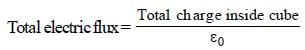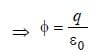31 Years NEET Previous Year Questions: Electric Charges & Fields - Question 7

The formation of a dipole is due to two equaland dissimilar point charges placed at a

Detailed Solution for 31 Years NEET Previous Year Questions: Electric Charges & Fields - Question 7

Dipole is formed when two equal and unlike charges are placed at a short distance.

31 Years NEET Previous Year Questions: Electric Charges & Fields - Question 8

From a point charge, there is a fixed point A. At A, there is an electric field of 500 V/m and potential difference of 3000 V. Distance between point charge and A will be 

Detailed Solution for 31 Years NEET Previous Year Questions: Electric Charges & Fields - Question 8

Given : Electric field (E) = 500 V/m
and potential difference (V) = 3000 V.
We know that electric field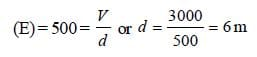[where d = Distance between point charge
and A]

31 Years NEET Previous Year Questions: Electric Charges & Fields - Question 9

A hollow insulated conduction sphere is given a positive charge of 10 μC. What will be the electric field at the centre of the sphere if its radius is 2 metres? 

Detailed Solution for 31 Years NEET Previous Year Questions: Electric Charges & Fields - Question 9

Charge resides on the outer surface of a conducting hollow sphere of radius R. We consider a spherical surface of radius r < R.
By Gauss's theorem,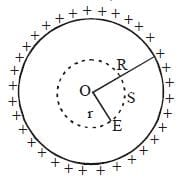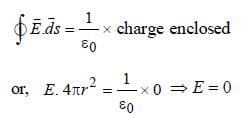i.e. electric field inside a hollow sphere is zero.

31 Years NEET Previous Year Questions: Electric Charges & Fields - Question 10

A particle of mass m and charge q is placed atrest in a uniform electric field E and then released.The kinetic energy attained by the particle after moving a distance y is 

Detailed Solution for 31 Years NEET Previous Year Questions: Electric Charges & Fields - Question 10

K.E. = Force × distance = qE.y

31 Years NEET Previous Year Questions: Electric Charges & Fields - Question 11

A point Q lies on the perpendicular bisector of an electrical dipole of dipole moment p. If the distance of Q from the dipole is r (much larger than the size of the dipole), then the electric field at Q is proportional to 

Detailed Solution for 31 Years NEET Previous Year Questions: Electric Charges & Fields - Question 11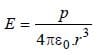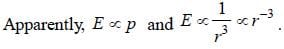31 Years NEET Previous Year Questions: Electric Charges & Fields - Question 12

When air is replaced by a dielectric medium of force constant K, the maximum force of attraction between two charges, separated by a distance 

Detailed Solution for 31 Years NEET Previous Year Questions: Electric Charges & Fields - Question 12

in air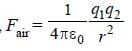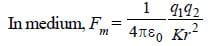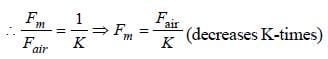31 Years NEET Previous Year Questions: Electric Charges & Fields - Question 13

A semi-circular arc of radius ‘a’ is charged uniformly and the charge per unit length is λ. The electric field at the centre of this arc is 

Detailed Solution for 31 Years NEET Previous Year Questions: Electric Charges & Fields - Question 13

λ = linear charge density;
Charge on elementary portion dx = λ dx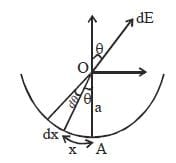Electric field at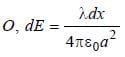Horizontal electric field, i.e., perpendicular to AO, will be cancelled.
Hence, net electric field = addition of all electrical fields in direction of AO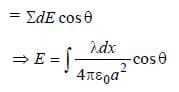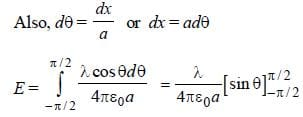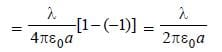31 Years NEET Previous Year Questions: Electric Charges & Fields - Question 14

A charge Q is placed at the corner of a cube, but is completely inside the cube.The electric flux through all the six faces of the cube is 

Detailed Solution for 31 Years NEET Previous Year Questions: Electric Charges & Fields - Question 14

According to Gauss's theorem, electric flux through a closed surface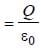where q is charge enclosed by the surface.

31 Years NEET Previous Year Questions: Electric Charges & Fields - Question 15

The electric intensity due to a dipole of length 10 cm and having a charge of 500 μC, at a point on the axis at a distance 20 cm from one of the charges in air, is 

Detailed Solution for 31 Years NEET Previous Year Questions: Electric Charges & Fields - Question 15

Given : Length of the dipole (2l) =10cm
= 0.1m or l = 0.05 m
Charge on the dipole (q) = 500 μC = 500 × 10–6 C and distance of the point on the axis from the mid-point of the dipole (r) = 20 + 5 = 25 cm = 0.25 m. We know that the electric field intensity due to dipole on the given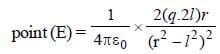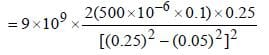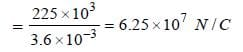31 Years NEET Previous Year Questions: Electric Charges & Fields - Question 16

If a dipole of dipole moment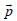is placed in a uniform electric field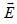, then torque acting on it is given by       

Detailed Solution for 31 Years NEET Previous Year Questions: Electric Charges & Fields - Question 16

Given : Dipole moment of the dipole =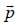and
uniform electric field =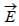We know that dipole moment (p) = q.a (where q is the charge and a is dipole length). And when a dipole of dipole momentis placed in a uniform electric field, then Torque (τ) = Either force × Perpendicular distance between the two forces = qaEsinθ or τ = pE sin θ or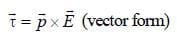31 Years NEET Previous Year Questions: Electric Charges & Fields - Question 17

A charge QμC is placed at the centre of a cube, the flux coming out from any surface will be

Detailed Solution for 31 Years NEET Previous Year Questions: Electric Charges & Fields - Question 17

Total flux out of all six faces as per Gauss's
theorem should be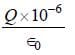Therefore, flux coming out of each face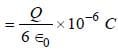31 Years NEET Previous Year Questions: Electric Charges & Fields - Question 18

An electron is moving round the nucleus of a hydrogen atom in a circular orbit of radius r. The Coulomb force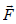between the two is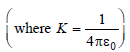Detailed Solution for 31 Years NEET Previous Year Questions: Electric Charges & Fields - Question 18

Charges (–e) on electron and (e) on proton exert a force of attraction given by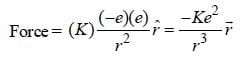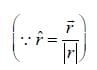Note : Magnitude of Coulomb force is given by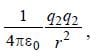but in vector form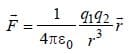31 Years NEET Previous Year Questions: Electric Charges & Fields - Question 19

A charge q is located at the centre of a cube. The electric flux through any face is 

Detailed Solution for 31 Years NEET Previous Year Questions: Electric Charges & Fields - Question 19

Cube has 6 faces. Flux through any face is given by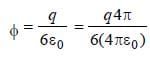31 Years NEET Previous Year Questions: Electric Charges & Fields - Question 20

A square surface of side L metres is in the plane of the paper. A uniform electric field(volt / m), also in the plane of the paper, is limited only to the lower half of the square surface (see figure). The electric flux in SI units associated with the surface is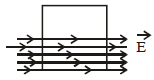Detailed Solution for 31 Years NEET Previous Year Questions: Electric Charges & Fields - Question 20

Flux =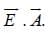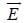is electric field vector &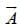is area vector

Here, angle between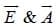is 90º.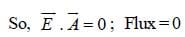31 Years NEET Previous Year Questions: Electric Charges & Fields - Question 21

An electric dipole of moment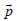is lying along a uniform electric field. The work done in rotating the dipole by 90° is

Detailed Solution for 31 Years NEET Previous Year Questions: Electric Charges & Fields - Question 21

Work done in rotating a dipole = pE (1 – cos θ)

If θ = 90º, work done = pE (1 – 0) = pE

31 Years NEET Previous Year Questions: Electric Charges & Fields - Question 22

A hollow cylinder has a charge q coulomb within it. If φ is the electric flux in units of voltmeter associated with the curved surface B, the flux linked with the plane surface A in units of voltmeter will be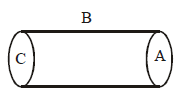Detailed Solution for 31 Years NEET Previous Year Questions: Electric Charges & Fields - Question 22

According to Gauss Law state that the net electric through the closed surface {Gaussian's surface} is equal to net charges inside the surface divided by ∈₀
Here, Let flux on A , B and C surfaces are ФA, ФB, ФC
Then, Фnet = ФA + ФB + ФC = q/∈₀
We see that , ФA = ФC and ФB = Ф
So, 2ФA + Ф = q/∈₀
ФA = 1/2(q/∈₀ - Ф)
Hence, the electric flux through plane surface is 1/2(q/∈₀ - Ф)

31 Years NEET Previous Year Questions: Electric Charges & Fields - Question 23

Three point charges +q, –q and +q are placed at points (x = 0, y = a, z = 0), (x = 0, y = 0, z = 0) and (x = a, y = 0, z = 0) respectively. The magnitude and direction of the electric dipole moment vector of this charge assembly are 

Detailed Solution for 31 Years NEET Previous Year Questions: Electric Charges & Fields - Question 23

Three point charges +q, –2q and +q are placed at points B (x = 0, y = a, z = 0), O (x = 0, y = 0, z = 0) and A(x = a, y = 0, z = 0)
The system consists of two dipole moment vectors due to (+q and –q) and again due to (+q and –q) charges having equal magnitudes qa units – one along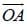and other along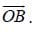Hence, net dipole moment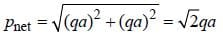along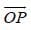at an angle 45° with positive X-axis.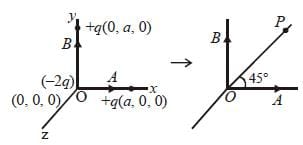31 Years NEET Previous Year Questions: Electric Charges & Fields - Question 24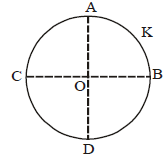A thin conducting ring of radius R is given a charge + Q. The electric field at the centre O of the ring due to the charge on the part AKB of the ring is E. The electric field at the centre due to the charge on the part ACDB of the ring is

Detailed Solution for 31 Years NEET Previous Year Questions: Electric Charges & Fields - Question 24

By the symmetry of the figure, the electric fields at O due to the portions AC and BD are equal in magnitude and opposite in direction. So, they cancel each other.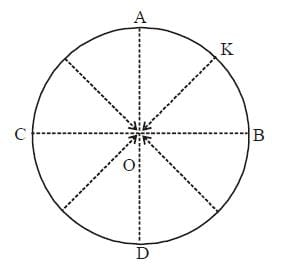Similarly, the field at O due to CD and AKB are equal in magnitude but opposite in direction. Therefore, the electric field at the centre due to the charge on the part ACDB is E along OK.

31 Years NEET Previous Year Questions: Electric Charges & Fields - Question 25

The mean free path of electrons in a metal is 4 × 10–8 m. The electric field which can give on an average 2 eV energy to an electron in the metal will be in units of V/m   

Detailed Solution for 31 Years NEET Previous Year Questions: Electric Charges & Fields - Question 25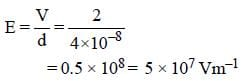31 Years NEET Previous Year Questions: Electric Charges & Fields - Question 26

Two positive ions, each carrying a charge q, are separated by a distance d. If F is the force of repulsion between the ions, the number of electrons missing from each ion will be (e being the charge of an electron) 

Detailed Solution for 31 Years NEET Previous Year Questions: Electric Charges & Fields - Question 26

Let n be the number of electrons missing.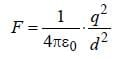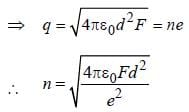31 Years NEET Previous Year Questions: Electric Charges & Fields - Question 27

A square surface of side L meter in the plane of the paper is placed in a uniform electric field E (volt/m) acting along the same plane at an angle θ with the horizontal side of the square as shown in Figure. The electric flux linked to the surface, in units of volt. m, is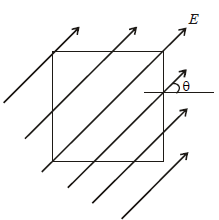Detailed Solution for 31 Years NEET Previous Year Questions: Electric Charges & Fields - Question 27

Electric flux, φ = EA cos θ , where θ = angle between E and normal to the surface.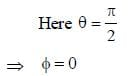31 Years NEET Previous Year Questions: Electric Charges & Fields - Question 28

The electric field at a distance 3R/2 from the centre of a charged conducting spherical shell of radius R is E. The electric field at a distance R/2  from the centre of the sphere is

Detailed Solution for 31 Years NEET Previous Year Questions: Electric Charges & Fields - Question 28

Electric field at a point inside a charged conducting spherical shell is zero.

31 Years NEET Previous Year Questions: Electric Charges & Fields - Question 29

A charge Q is enclosed by a Gaussian spherical surface of radius R. If the radius is doubled, then the outward electric flux will 

Detailed Solution for 31 Years NEET Previous Year Questions: Electric Charges & Fields - Question 29

By Gauss’s theorem,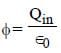Thus, the net flux depends only on the charge enclosed by the surface. Hence, there will be no effect on the net flux if the radius of the surface is doubled

31 Years NEET Previous Year Questions: Electric Charges & Fields - Question 30

The electric potential V at any point (x, y, z), all in meters in space is given by V = 4x2 volt. The electric field at the point (1, 0, 2) in volt/meteris [2011M]

Detailed Solution for 31 Years NEET Previous Year Questions: Electric Charges & Fields - Question 30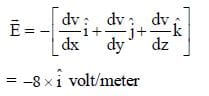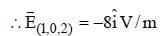31 Years NEET Previous Year Questions: Electric Charges & Fields - Question 31

Three charges, each +q, are placed at the corners of an isosceles triangle ABC of sides BC and AC, 2a. D and E are the mid points of BC and CA. The work done in taking a charge Q from D to E is   [2011 M]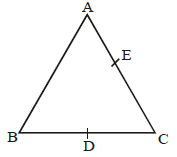Detailed Solution for 31 Years NEET Previous Year Questions: Electric Charges & Fields - Question 31

AC = BC
VD = VE
We have,
W = Q (VE – VD)

⇒W=0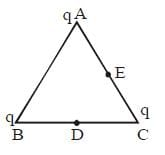31 Years NEET Previous Year Questions: Electric Charges & Fields - Question 32

An electric dipole of moment ´p´ is placed in an electric field of intensity ´E´. The dipole acquires a position such that the axis of the dipole makes an angle θ with the direction of the field. Assuming that the potential energy of the dipole to be zero when = 90°, the torque and the potential energy of the dipole will respectively be :

Detailed Solution for 31 Years NEET Previous Year Questions: Electric Charges & Fields - Question 32

The torque on the dipole is given as τ = pE sin θ
The potential energy of the dipole in the electric field is given as
U = – pE cos θ

31 Years NEET Previous Year Questions: Electric Charges & Fields - Question 33

What is the flux through a cube of side 'a' if a point charge of q is at one of its corner :       

Detailed Solution for 31 Years NEET Previous Year Questions: Electric Charges & Fields - Question 33

Eight identical cubes are required to arrange
so that this charge is at centre of the cube formed so flux.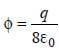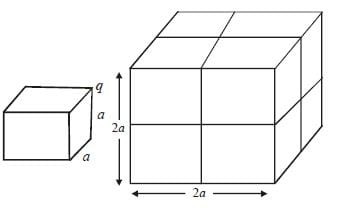31 Years NEET Previous Year Questions: Electric Charges & Fields - Question 34

Two metallic spheres of radii 1 cm and 3 cm are given charges of –1×10–2 C and 5×10–2 C, respectively. If these are connected by a conducting wire, the final charge on the bigger sphere is : [2012M]

Detailed Solution for 31 Years NEET Previous Year Questions: Electric Charges & Fields - Question 34

At equilibrium potential of both sphere becomes same if charge of sphere one x
and other sphere Q – x then
where Q = 4 × 10–2 C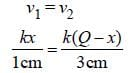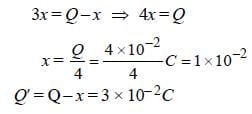31 Years NEET Previous Year Questions: Electric Charges & Fields - Question 35

Two pith balls carrying equal charges are suspended from a common point by strings of equal length. The equilibrium separation
between them is r. Now the strings are rigidly clamped at half the height. The equilibrium separation between the balls now become
[NEET 2013]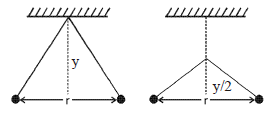Detailed Solution for 31 Years NEET Previous Year Questions: Electric Charges & Fields - Question 35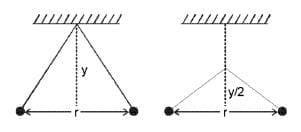From figure,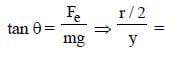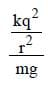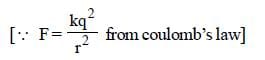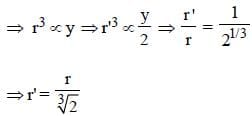31 Years NEET Previous Year Questions: Electric Charges & Fields - Question 36

A charge ‘q’ is placed at the centre of the line joining two equal charges ‘Q’. The system of the three charges will be in equilibrium if ‘q’ is equal to

Detailed Solution for 31 Years NEET Previous Year Questions: Electric Charges & Fields - Question 36

The system of three charges will be in equilibrium.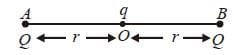For this, force between charge at A and B + force between charge at point O and either at A or B is zero.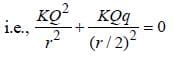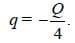31 Years NEET Previous Year Questions: Electric Charges & Fields - Question 37

An electric dipole of dipole moment p is aligned parallel to a uniform electric field E. The energy required to rotate the dipole by 90° is   [NEET Kar. 2013]

Detailed Solution for 31 Years NEET Previous Year Questions: Electric Charges & Fields - Question 37

When electric dipole is aligned parallel θ = 0° and the dipole is rotated by 90° i.e.,
θ = 90°.
Energy required to rotate the dipole W = Uf – Ui = (–pE cos 90°) – (–pE cos 0°)
= pE.

## Physics Class 12

157 videos|452 docs|213 tests
 Use Code STAYHOME200 and get INR 200 additional OFF Use Coupon Code
Information about 31 Years NEET Previous Year Questions: Electric Charges & Fields Page
In this test you can find the Exam questions for 31 Years NEET Previous Year Questions: Electric Charges & Fields solved & explained in the simplest way possible. Besides giving Questions and answers for 31 Years NEET Previous Year Questions: Electric Charges & Fields, EduRev gives you an ample number of Online tests for practice

## Physics Class 12

157 videos|452 docs|213 tests

### How to Prepare for NEET

Read our guide to prepare for NEET which is created by Toppers & the best Teachers